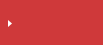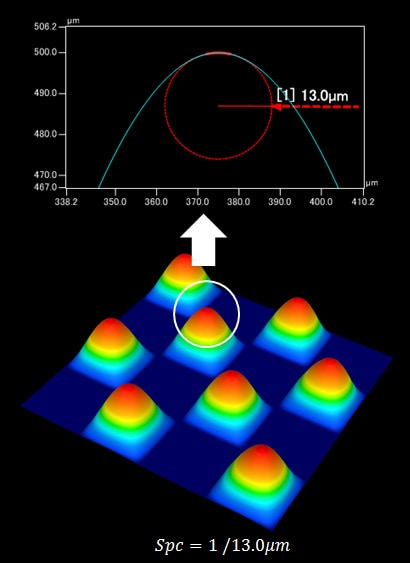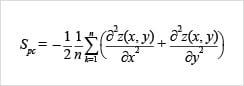>  What is area roughness?  >  Area Roughness Parameters

# Spc (Arithmetic Mean Peak Curvature) | Area Roughness Parameters

## Description of surface texture parameters in ISO 25178

• Height
• Spatial
• Hybrid
• Functional
• Functional volume
• FeatureFind from the parameter list

## Spc (Arithmetic Mean Peak Curvature)

Spc (arithmetic mean peak curvature) represents the arithmetic mean of the principal curvature of the peaks on the surface. A smaller value indicates that the points of contact with other objects have rounded shapes; a larger value indicates that the points of contact with other objects have pointed shapes.## Find from the parameter list

Height Sa (arithmetical mean height) Sz (Maximum height) Sq (Root mean square height) Ssk (Skewness) Sku (Kurtosis) Sp (Maximum peak height) Sv (Maximum pit height) Sal (Auto-correlation length) / Str (Texture aspect ratio) Std* (Texture direction) Sdq (Root mean square gradient) Sdr (Developed interfacial area ratio) Smr(c) Areal material (bearing area) ratio Smc(mr) Inverse areal material ratio Sk(Core height) Spk (Reduced peak height) Svk (reduced dale height (reduced valley depth)) Smr (Peak material portion) Smr2 (Valley material portion) Sxp (Peak extreme height) Vvv (Dale void volume) Vvc (Core void volume) Vmp (Peak material volume) Vmc (Core material volume) Spd (Density of peaks) Spc (Arithmetic mean peak curvature) S10z / S5p / S5v / Sda(c) / Sha(c) / Sdv(c) / Shv(c)